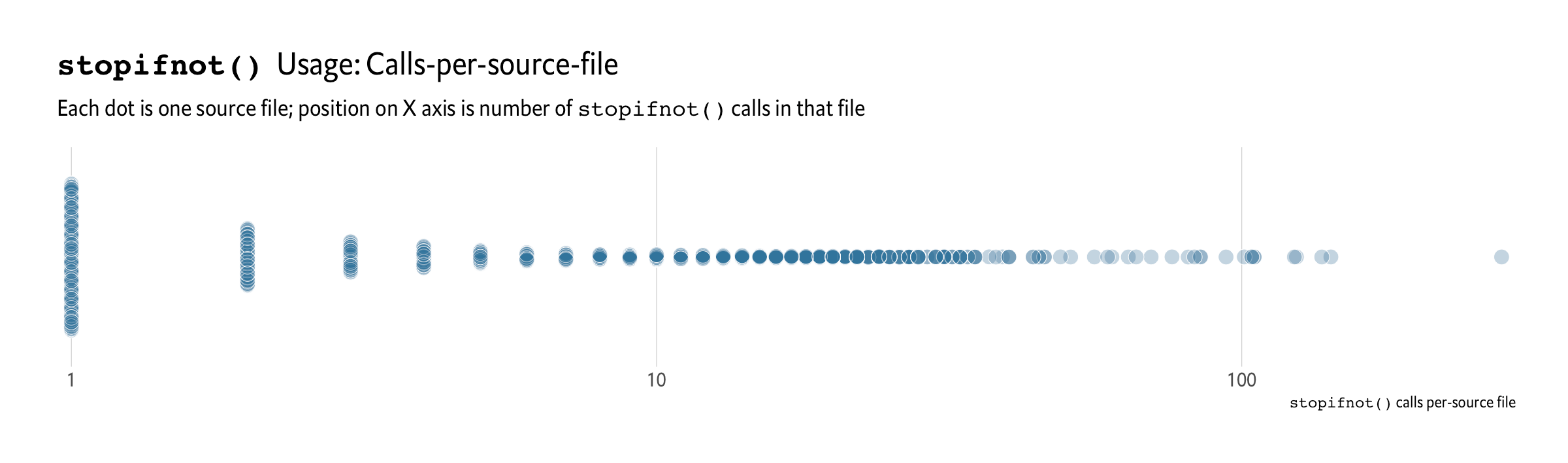# Mining R 4.0.0 Changelog for Nuggets of Gold: #1 stopifnot()

R 4.0.0 has been out for a while, now, and — apart from a case where `merge()` was slower than dirt — it’s been really stable for at least me (I use it daily on macOS, Linux, and Windows). Sure, it came with some headline-grabbing features/upgrades, but I’ve started looking at what other useful nuggets might be in the changelog and decided to blog them as I find them.

Today’s nugget is the venerable `stopifnot()` function which was significantly enhanced by this PR by Neil Fultz.

Prior to R 4.0.0, if you wanted to use `stopifnot()` to perform some input validation (a.k.a. — in this case — [assertions])(https://en.wikipedia.org/wiki/Assertion_(software_development)) you’d do something like this (I’m borrowing from Neil’s example):

``````some_ƒ <- function(alpha, gradtol, steptol, interlim) {

stopifnot(
(is.numeric(alpha)),
(length(alpha) == 1),
(alpha > 0),
(alpha < 1),
(is.numeric(steptol)),
(length(steptol) == 1),
(steptol > 0),
(is.numeric(interlim)),
(length(interlim) == 1),
(interlim > 0)
)

message("Do something awesome")

}
``````

When run with acceptable inputs we get:

``````some_ƒ(0.5, 3, 10, 100)
## Do something awesome
``````

But, when run with something out of kilter:

``````some_ƒ("a", 3, 10, 100)
##  Error in some_ƒ("a", 3, 10, 100) : (is.numeric(alpha)) is not TRUE
``````

we get a semi-useful, but somewhat unfriendly message back. Sure, it points to the right expression, but we’re supposed to be the kinder, friendlier data science (and general purpose) language who cares a bit more about our users. To that end, many folks switch to doing something like this:

``````some_ƒ <- function(alpha, gradtol, steptol, interlim) {

if (!is.numeric(alpha))   { stop('Error: alpha should be numeric') }
if (length(alpha) != 1)   { stop('Error: alpha should be a single value'); }
if (alpha < 0)            { stop('Error: alpha is negative'); }
if (alpha > 1)            { stop('Error: alpha is greater than one'); }
if (length(gradtol) != 1) { stop('Error: gradtol should be a single value'); }
if (!is.numeric(steptol)) { stop('Error: steptol should be numeric') }
if (length(steptol) != 1) { stop('Error: steptol should be a single value'); }
if (steptol <= 0)         { stop('Error: steptol should be positive'); }
if (!is.numeric(iterlim)) { stop('Error: iterlim should be numeric') }
if (length(iterlim) != 1) { stop('Error: iterlim should be a single value'); }
if (iterlim <= 0)         { stop('Error: iterlim should be positive'); }

message("Do something awesome")

}
``````

which results in:

``````some_ƒ("a", 3, 10, 100)
##  Error in some_ƒ("a", 3, 10, 100) : Error: alpha should be numeric
``````

(you can make even better error messages than that).

Neal thought there had to be a better way, and made one! The `...` expressions can be named and those names will become the error message:

``````some_ƒ <- function(alpha, gradtol, steptol, interlim) {

stopifnot(
'alpha should be numeric'          = (is.numeric(alpha)),
'alpha should be a single value'   = (length(alpha) == 1),
'alpha is negative'                = (alpha > 0),
'alpha is greater than one'        = (alpha < 1),
'steptol should be numeric'        = (is.numeric(steptol)),
'steptol should be a single value' = (length(steptol) == 1),
'steptol should be positive'       = (steptol > 0),
'iterlim should be numeric'        = (is.numeric(interlim)),
'iterlim should be a single value' = (length(interlim) == 1),
'iterlim should be positive'       = (interlim > 0)
)

message("Do something awesome")

}

some_ƒ("a", 3, 10, 100)

##  Error in some_ƒ("a", 3, 10, 100) : alpha should be numeric
``````

Way easier to write and way more respectful to the caller.

### Gratuitous Statistics

CRAN has ~2,600 packages that use `stopifnot()` in their package `/R/` code with the following selected distributions (charts are all log10 scale):Here are the packages with 50 or more files using `stopifnot()`:

``````   pkg              n
<chr>        <int>
1 spatstat       252
2 pracma         145
3 QuACN           80
4 raster          74
5 spdep           61
6 lavaan          54
7 surveillance    53
8 copula          50
``````Here are the packages with one or more files that have 100 or more calls to `stopifnot()` in them:

``````   pkg                 fil                            ct
<chr>               <chr>                       <int>
1 ff                  ordermerge.R                  278
2 OneArmPhaseTwoStudy zzz.R                         142
3 bit64               integer64.R                   137
4 updog               rflexdog.R                    124
5 RNetCDF             RNetCDF.R                     123
6 Rlda                rlda.R                        105
7 aster2              transform.R                   105
9 georob              georob_exported_functions.R   104
10 bit64               highlevel64.R                 101
``````

O_O That’s quite a bit of checking!

### FIN

If you’re working on switching to R 4.0.0 or have switched, this and many other new features await! Drop a note in the comments with your favorite new feature (or, even better, a link to a blog post on said feature!).

As I get time to dig out some more nuggets I’ll add more posts to this series.Amazon Author Page

## 1 Comment →Mining R 4.0.0 Changelog for Nuggets of Gold: #1 stopifnot()

This site uses Akismet to reduce spam. Learn how your comment data is processed.